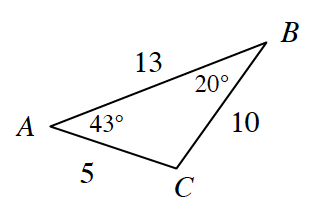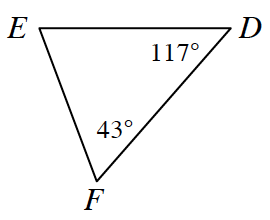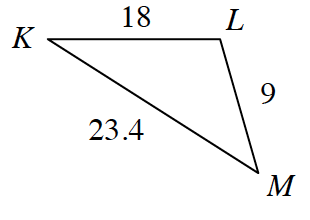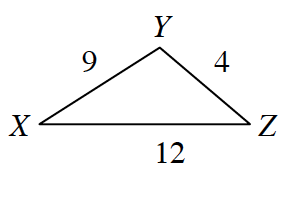### Home > GC > Chapter 11 > Lesson 11.1.4 > Problem11-54

11-54.

For each triangle below, decide if it is similar to the triangle at right. If it is similar, justify your conclusion and complete the similarity statement $ΔABC ∼ Δ\text{___}$. If the triangle is not similar, explain how you know. Assume that the diagrams are not drawn to scale.1.Find the measures of the unknown angles.
Does this prove they are similar?

1.$ΔABC~ΔMLK\left(\text{SSS}\sim\right)$

1.Is there a zoom factor?